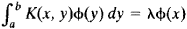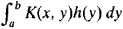# Eigenfunction

(redirected from Eigen function)
Also found in: Dictionary, Medical.
Related to Eigen function: eigenfunction expansion

## Eigenfunction

One of the solutions of an eigenvalue equation. A parameter-dependent equation that possesses nonvanishing solutions only for particular values (eigenvalues) of the parameter is an eigenvalue equation, the associated solutions being the eigenfunctions (sometimes eigenvectors). In older usage the terms characteristic equation and characteristic values (functions) are common. Eigenvalue equations appear in many contexts, including the solution of systems of linear algebraic equations (matrix equations), differential or partial differential equations, and integral equations. The importance of eigenfunctions and eigenvalues in applied mathematics results from the widespread applicability of linear equations as exact or approximate descriptions of physical systems. However, the most fundamental application of these concepts is in quantum mechanics where they enter into the definition and physical interpretation of the theory. Only linear eigenvalue equations will be discussed. See Eigenvalue (quantum mechanics), Energy level (quantum mechanics), Quantum mechanics

McGraw-Hill Concise Encyclopedia of Physics. © 2002 by The McGraw-Hill Companies, Inc.
The following article is from The Great Soviet Encyclopedia (1979). It might be outdated or ideologically biased.

## Eigenfunction

a concept of mathematical analysis. The solution of many problems in mathematical physics—for example, in the theory of vibrations and the theory of heat conduction—requires the finding of nontrivial solutions of homogeneous linear differential equations L(y) = Xy where the solutions satisfy particular boundary conditions. Such solutions are called ei-genfunctions of the problem, and the corresponding values of λ are called eigenvalues.

If a differential equation with appropriate boundary conditions is self-adjoint, then its eigenvalues are real, and the eigenfunctions corresponding to distinct eigenvalues are orthogonal. If the differential equation is considered on a finite interval and its coefficients have no singularities on the interval, then the set of eigen-functions is countable—that is, the problem has a discrete spectrum. If we know the eigenfunctions and the corresponding eigenvalues, then, under certain conditions, we can obtain a solution of the problem in the form of a series of eigenfunctions. Suppose, however, the equation is considered on an infinite interval or its coefficients have singularities (for example, the coefficient of the derivative of highest order takes on the value 0). A continuum of eigenfunctions may then exist, and expansion in the form of an integral with respect to the eigenfunctions is obtained instead of a series expansion. Such an integral is analogous to a Fourier integral representation. In this case, the problem is said to have a continuous spectrum. Many special functions, such as orthogonal polynomials, serve as the eigenfunctions of some equations.

In the theory of integral equations, a function that for some value of X satisfies the equationis called an eigenfunction of the kernel K(x, y). Any symmetric continuous kernel has an eigenfunction. In this case, any function representable in the formcan be expanded in a series of eigenfunctions. If the kernel has singularities or is defined in an infinite domain, then a continuous spectrum may also arise.

Eigenfunctions can be defined most generally as the eigenvectors of linear operators in linear function spaces. In quantum mechanics, the eigenfunctions of an operator corresponding to some physical quantity correspond to those states of a system in which the given physical quantity has a definite value.

Eigenfunctions are sometimes also called proper functions or characteristic functions.

## eigenfunction

[′ī·gən‚fəŋk·shən]
(mathematics)
Also known as characteristic function.
An eigenvector for a linear operator on a vector space whose vectors are functions. Also known as proper function.
A solution to the Sturm-Liouville partial differential equation.
McGraw-Hill Dictionary of Scientific & Technical Terms, 6E, Copyright © 2003 by The McGraw-Hill Companies, Inc.
References in periodicals archive ?
A stop band rejection better than 54 dB is obtained using eigen function [H.sub.12].
This article discusses the various requirements of SAW filters used in the IF stages of wireless handsets designed for use with different standards and presents several design options using eigen functions to realize the SAW filters.
The increasing demand for smaller filter sizes for wireless applications emphasizes that the impulse duration of the transfer function should be kept to a minimum, which, in turn, dictates that a minimum number of possible eigen functions be employed to realize the desired response.
Even and odd cosine series functions in the time domain that are used as eigen functions for SAW filter realization have been described. As the number of terms (N) in the functions increase, the side lobe levels decrease and the main lobe width increases.
The procedure uses two eigen functions to realize the input IDT transfer function.
To improve the stop band rejection, higher order eigen functions must be employed.
Two parameters, [Q.sub.1] and [Q.sub.2], are used to inter-relate the spacing between the eigen functions, their impulse duration and the bandwidth of sinc response.
For a given impulse duration T, [Q.sub.1] determines the separation B between the two eigen functions and [Q.sub.2] determines the null-to-null bandwidth [B.sub.o] of the output IDT transfer function.
The filter incorporates only two eigen functions ([H.sub.31]) placed 700 kHz apart on either side of the center frequency.
We consider the Eigen functions of the differential operator.
The choice of trial functions to be considered are the Eigen functions of the differential operator which has been discussed for the solution of Poisson's equation in the T-shaped geometry.

Site: Follow: Share:
Open / Close